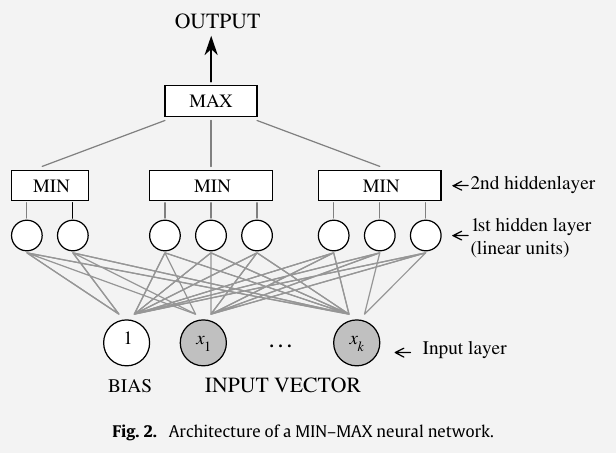# Monotone Multi-Layer Perceptron

##### Posted on Jul 04, 2022

This note is for monotonic Multi-Layer Perceptron Neural network, and the references are from the R package monmlp.

## Zhang and Zhang (1999)

Zhang, H., & Zhang, Z. (1999). Feedforward networks with monotone constraints. IJCNN’99. International Joint Conference on Neural Networks. Proceedings (Cat. No.99CH36339), 3, 1820–1823 vol.3.

The proposed network structure is similar to that of an ordinary multilayer perceptron except that the weights $w_i$ are replaced by $e^{w_i}$.

A single neuron output is defined as

$z_j = g(a_j)\,, \qquad a_j=b_j+\sum_ie^{w_{ij}}z_i$

It can be shown that $y$ is a always an increasing function of $x$.

## Lang (2005)

Lang, B. (2005). Monotonic Multi-layer Perceptron Networks as Universal Approximators. In W. Duch, J. Kacprzyk, E. Oja, & S. Zadrożny (Eds.), Artificial Neural Networks: Formal Models and Their Applications – ICANN 2005 (Vol. 3697, pp. 31–37). Springer Berlin Heidelberg.

A fully connected multi-layer perceptron network (MLP) with $I$ inputs, a first hidden layer with $H$ nodes, a second hidden layer with $L$ nodes and a single output is defined by

\begin{align*} \hat y(\bfx) &= w_b + \sum_{l=1}^L w_l\tanh (w_{b,l} + \sum_{h=1}^H \tanh(w_{b,h}+\sum_{i=1}^I w_{hi}x_i))\\ &=w_b + \sum_{l=1}^L w_l\tanh (w_{b,l} + \sum_{h=1}^H \theta_2)\\ &=w_b + \sum_{l=1}^L w_l\theta_1\\ \end{align*}

MLP ensures a monotonically increasing behavior with respect to the input $x_j\in \bfx$ if

$\frac{\partial \hat y}{\partial x_j} = \sum_{l=1}^Lw_l\cdot (1-\theta_1^2)\sum_{h=1}^Hw_{lh}(1-\theta_2^2)w_{hj} \ge 0$

The derivative of a hyperbolic tangent is always positive. For this reason, a sufficient condition for a monotonicity increasing behavior for the input dimension $j$ is defined as

$w_l \cdot w_{lh} \cdot w_{hj} \ge 0 \forall l, h\,.$

## Minin et al. (2010)

Minin, A., Velikova, M., Lang, B., & Daniels, H. (2010). Comparison of universal approximators incorporating partial monotonicity by structure. Neural Networks, 23(4), 471–475.Let $R$ denote the number of nodes in the second hidden layer, which equals the number of groups in the first hidden layer. The outputs of the groups are denoted by $g_1,\ldots,g_R$. Let $h_r$ denote the number of hyperplanes within group $r, r=1,2,\ldots, R$. The output at group $r$ is defined by

$g_r(\bfx) = \min_j(w_{r,j}\cdot x + \theta_{(r, j)}), 1\le j\le h_r\,,$

the final output $\hat y(\bfx)$ of the network for an input $\bfx$ is

$\hat y(\bfx) = \max_r g_r(\bfx)\,.$

Published in categories Note